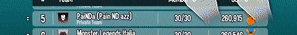• D

### Damage formula

Post count: 128
Reputation: 98
Joined:

First, since it seems like a lot of people don't know that, floor(number)=number not including the decimal places. For example, floor(2.94)=2.
So I think that I found an exact damage formula not including level difference, elemental advantage/weakness and damage boosts/reductions(though i assume that it's floor(damage without modifiers*modifiers), not sure yet).
If you think you can prove me wrong, reply with the following information:
-level of the attacker
-runes of your attacker(as well as team strength runes if you have that)
-the power of the move
-the attacker
-calculated damage
-actual damage
Anyway, the formula is:
floor(floor(floor(runeMult*basePower)*movePower/50)*(level+5)/50), where basePower is the power of your monster with no runes applied and runeMult is the sum of all percentages of the strength runes that affect your monsters divided by 100, plus 1.
For example, let's say we had a level 130 igursus with a level 5 and a level 4 strength rune use Etna against a level 130 opponent.
The level 5 strength rune is +48% damage and the level 4 strength rune is +36% damage. The sum of the percentages is 84, divided by 100 is 0.84, plus 1 is 1.84.
The base power of Igursus at level 130 is 4410.
So, let's calculate:
floor(floor(floor(1.84*4410)*65/50)*135/50) = floor(floor(floor(8114.4)*1.3)*2.7) = floor(floor(8114*1.3)*2.7) = floor(floor(10548.2)*2.7) = floor(10548*2.7) = floor(28479.6)=28479 damage.
I also made this into a miniature excel sheet, which you can make a copy of and mess around with by going to File->Make a copy.

• ?

### Damage formula

Post count: 0
Reputation: 0
Joined:

Thank you! (ML NotPro)

•### Damage formula

Post count: 4495
Reputation: 1646
Joined:

@Carlos can you take a lookJoin Discord, Official Monster Legend: https://discord.gg/vnWasWa and Monsters Legend Community Discord: discord.gg/kqkG6M8 to talk about ML.
and checkout the unofficial Monster Legends Wiki https://monsterlegends.wikia.com/wiki/Monster_Legends_Wiki

• M

### Damage formula

Post count: 477
Reputation: 135
Joined:

Wow this is really well done. Bravo!

•### Damage formula

Post count: 3260
Reputation: 1305
Joined:

It was discussed heavily on old forum hope @sansot has a screenshots
but you can check
@C0ntr1v3d said in Who is arguably the best monster for dealing huge damage in one hit?:

@sansot

Basic_damage = (attackerLevel * 0.4 + 2) * skillPower * (attackerPower/1000)

Total_damage = (Basic_damage) (1 + RuneBonuses) * (1 + greaterLevelModifier) * elementEffect * attackModifiers

Where:

greaterLevelModifier = 0.02 * levelGreater BUT Limit 0 - ie cannot be negative. This means a lower level monster attacking a higher level monster has no penalty (value = zero).

elementEffect = 0.5 if weak, 1.0 if neutral, 1.5 if strong, 1.8 for Debilitating Shadows, Sticky Lava etc

attackModifiers are summed (e.g., Daze = -25%, Damage Reduction of -25%/-50%/-100%, increased damage of +50%, +100%, etc.)

RuneBonuses are expressed as a decimal (converting percent to decimal)

(The 0.02 greaterLevelModifier is actually more like 0.0199999985 but 0.02 is accurate enough)

and
@Rob-LeDrew said in Monster Stats Sortable List:

@C0ntr1v3d

Because I feed my monsters as high as their star level will allow, I worked out the coefficients for strength/health/speed and put them in a Google Sheet that I use to track everyone and try different combinations.

Here's the formula I use for Strength:

=SWITCH(L53,105,"0.04545454545",110,"0.09090909091",115,"0.1362237762",120,"0.1818181818",130,"0.2727272727","0")

Here's the formula I use for Life:

=SWITCH(L53,105,"0.1019759101",110,"0.2088872997",115,"0.3208113907",120,"0.437711182",130,"0.6863432532","0")

And here's the formula I use for speed:

=SWITCH(L53,105,"0.04545454545",110,"0.09090909091",115,"0.1362237762",120,"0.1818181818",130,"0.2727272727","0")

I calculate by taking the level 100 value from the Monster Wiki site and multiplying like so:

=round(sum((level_100_life*life_coefficient)+level_100_life))

For example, a level 100 Cloud has a life of 36,795. To calculate the life of a level 130 Cloud, I use:

(36,795 * 0.6863432532) + 36,795 = 62049

19 May 2013 - 4 October 2018• D

### Damage formula

Post count: 128
Reputation: 98
Joined:

@Haka-Taka Yes, I already knew about that first formula; my problem with them is that it isn't exact. Actually, if you remove all the floors from my formula, it becomes the same as the one you mentioned.
Anyway, let's calculate the damage the example Igursus deals with that formula:
4410*1.84*54*65/1000=28481.544
As you can see, the result is off by 2.544; my goal with this post was to find an exact formula.
Also, one thing I didn't mention in the post is that I didn't test this formula with levels not divisible by 5 and move powers not divisible by 5.

• D

### Damage formula

Post count: 128
Reputation: 98
Joined:

The second formula for life is also an approximation: Let's look at some things at level 105.
Rockantium at level 100 has 32572 life, and at level 105 he has 35983 life. The formula says that he has 35983.56 life, which can be transformed into 35983 only by rounding down.
Arch Knight at level 100 has 23524 life, and at level 105 he has 25923 life. The formula says that he has 25922.88 life, which gives 25922 after rounding down(which is required for the first example to work). That means the formula isn't exact. On the other hand, I think I have an exact formula:
=CEILING(L70*L70*(I70-IF(I70>38000,200+MOD(I70,100),100+MOD(I70,100)))/10000+IF(I70>38000,200+MOD(I70,100),100+MOD(I70,100)),1), where L70 is the level and I70 is life at level 100. Again, you can try to find a monster on which my formula doesn't work and report it to me(though i'm really confident that it works).

Posts 7Views 3530

Looks like your connection to Socialpoint Forums was lost, please wait while we try to reconnect.